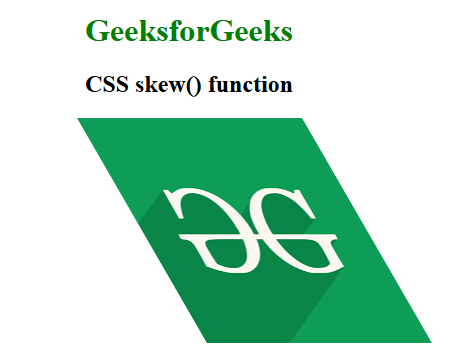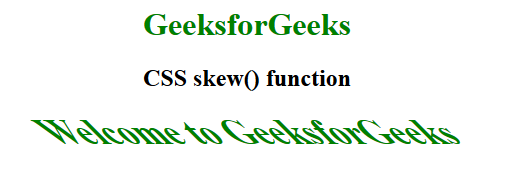# CSS skew() Function

The skew() function is an inbuilt function which is used to transform an element in the 2D plane. Skew an element means to pick a point and push or pull it in different directions.

Syntax:

`skew( ax )`

or

`skew( ax, ay )`

Parameters:

• ax: This parameter holds the angle representing the horizontal axis to distort an element.
• ay: This parameter holds the angle representing the vertical axis to distort an element. If it is not defined then it takes the default value zero. It means completely skew in x direction.

Below examples illustrate the skew() function in CSS:

Example 1:

## html

 `  ` `<``html``>  ` ` `  `<``head``>  ` `    ``<``title``>CSS skew() function  ` ` `  `    ``<``style``>  ` `        ``body { ` `            ``text-align:center; ` `        ``} ` `        ``h1 { ` `            ``color:green; ` `        ``} ` `        ``.skew_image { ` `            ``transform-origin: top left; ` `            ``transform: skew(30deg, 0); ` `        ``} ` `    ``  ` `  ` ` `  `<``body``>  ` `    ``<``h1``>GeeksforGeeks ` `    ``<``h2``>CSS skew() function ` `     `  `    ``<``img` `class``=``"skew_image"` `src``=  ` `"https://media.geeksforgeeks.org/wp-content/cdn-uploads/20190710102234/download3.png"` `        ``alt``=``"GeeksforGeeks logo"``>  ` `  ` ` `  `     `

Output:Example 2:

## html

 `  ` `<``html``>  ` ` `  `<``head``>  ` `    ``<``title``>CSS skew() function  ` ` `  `    ``<``style``>  ` `        ``body { ` `            ``text-align:center; ` `        ``} ` `        ``h1 { ` `            ``color:green; ` `        ``} ` `        ``.GFG { ` `            ``font-size:35px; ` `            ``font-weight:bold; ` `            ``color:green; ` `            ``transform: skew(45deg); ` `        ``} ` `    ``  ` `  ` ` `  `<``body``>  ` `    ``<``h1``>GeeksforGeeks ` `    ``<``h2``>CSS skew() function ` `     `  `    ``<``div` `class``=``"GFG"``>Welcome to GeeksforGeeks  ` `  ` ` `  `     `

Output:Supported Browsers: The browsers supported by skew() function are listed below: